# Angles

Determine the interior angles of a rhombus with area 319.1 cm2 and perimeter 72 cm.

α =  80 °
β =  100 °
γ =  80 °
δ =  100 °

### Step-by-step explanation:

$\beta =18{0}^{\circ }-\alpha =10{0}^{\circ }$
$\gamma =\alpha =8{0}^{\circ }$
$\delta =\beta =10{0}^{\circ }$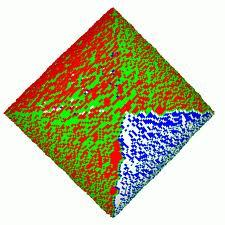We will be pleased if You send us any improvements to this math problem. Thank you!Tips to related online calculators
Do you want to convert length units?
Most natural application of trigonometry and trigonometric functions is a calculation of the triangles. Common and less common calculations of different types of triangles offers our triangle calculator. Word trigonometry comes from Greek and literally means triangle calculation.

#### You need to know the following knowledge to solve this word math problem:

We encourage you to watch this tutorial video on this math problem:

## Related math problems and questions:

• IS triangleCalculate interior angles of the isosceles triangle with base 40 cm and legs 22 cm long.
• Right triangleCalculate the missing side b and interior angles, perimeter, and area of ​​a right triangle if a=10 cm and hypotenuse c = 16 cm.
• Jewel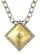Rhombus-shaped jewel has an area of 93 mm2 and the edge in long 13.2 mm. Calculate the size of the rhombus acute angle.
• ParallelogramCalculate area of the parallelogram ABCD as shown if |AB| = 19 cm, |BC| = 18 cm and angle BAD = 90°
• Triangle SASCalculate the triangle area and perimeter, if the two sides are 51 cm and 110 cm long and angle them clamped is 130 °.
• Parallelogram +ľ| AB | = 76cm, | BC | = 44cm, angle BAD = 30 °. Find the area of the parallelogram.
• Diagonals of the rhombus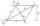Calculate height of rhombus whose diagonals are 12 cm and 19 cm.
• Regular 5-gonCalculate the area of the regular pentagon with side 7 cm.
• Rhombus A2p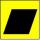Rhombus area is 13 cm2 and its height is 5cm long . Determine a perimeter of this rhombus.
• Triangle SAAThe triangle has one side long 71 m and its two internal angles is 60°. Calculate the perimeter and area of the triangle.
• Rhombus ABCDRhombus ABCD, |AC| = 90 cm, |BD| = 49 cm. Calculate the perimeter of the rhombus ABCD.
• A trapezoidA trapezoid with a base length of a = 36.6 cm, with angles α = 60°, β = 48° and the height of the trapezoid is 20 cm. Calculate the lengths of the other sides of the trapezoid.
• Maximum area of rhombusCalculate the interior angles at which equilateral rhombus has a maximum area.
• RhombusCalculate the perimeter and area of ​​a rhombus whose diagonals are 39 cm and 51 cm long.
• Space diagonal anglesCalculate the angle between the body diagonal and the side edge c of the block with dimensions: a = 28cm, b = 45cm and c = 73cm. Then, find the angle between the body diagonal and the plane of the base ABCD.
• ParallelogramFind the parallelogram's perimeter, where base a = 8 cm, height v = 3 cm, and angle alpha = 35° is the magnitude of the angle at vertex A.
• Which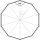Which of the following numbers most accurately area of a regular decagon with side s = 2 cm? (A) 9.51 cm2 (B) 20 cm2 (C) 30.78 cm2 (D) 31.84 cm2 (E) 32.90 cm2